# How To Filter The Data Based On The Selection Of Cell Using VBA In Microsoft Excel

In this article, you will learn how to filter the data based on the selection of cell.

You need to follow the below steps:

• Click on Developer tab
• From Code group, select Visual Basic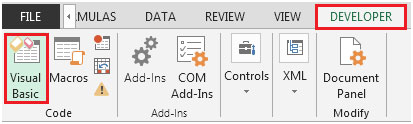Enter the following code in the current worksheet (sheet1 in our example)

Private Sub Worksheet_BeforeDoubleClick(ByVal Target As Range, Cancel As Boolean)

Dim xcolumn As Integer

Dim xvalue As String

xcolumn = ActiveCell.Column

xvalue = ActiveCell.Value

If Application.Intersect(ActiveCell, [headers]) Is Nothing Then

If ActiveCell.Value<> "" Then

ActiveSheet.Range("A:d").AutoFilter Field:=xcolumn, Criteria1:=xvalue

Cancel = True

End If

End If

End Sub

Private Sub Worksheet_SelectionChange(ByVal Target As Range)

Dim rownumber As Integer

rownumber = ActiveCell.Row

If Application.Intersect(ActiveCell, [headers]) Is Nothing Then

If ActiveCell.Value<> "" Then

Range("A1:D13").Interior.ColorIndex = xlNone

Range("A" &rownumber& ":D" &rownumber).Interior.ColorIndex = 6

End If

End If

End Sub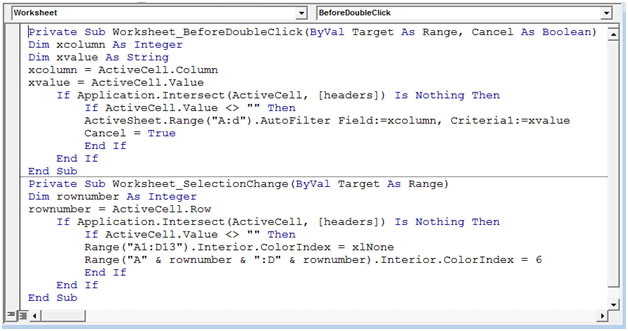Note: Headers in the above code represent named range for range A1:D1

You can simply select the range A1:D1 & type headers in the Name box or you can use Defined Name in Formulas tab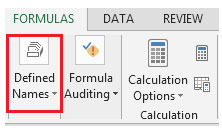• The BeforeDoubleClick event will get trigger every time the user hits double click on any cell & it will change filter down the search.
• If we want to filter the data by Product C then, we can easily double click on cell D4 to filter the search.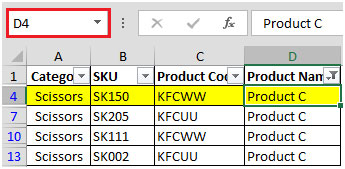In case we want to filter down the search by column C & Product KFCWW then, we will double click on cell C4 to narrow down the data.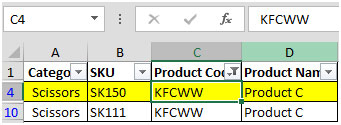• The SelectionChange event will get activated every time the user selects any cell & it will change the color from white to yellow. If active cell is empty then the code will not run.
• If we click on cell B3 then, the row will be highlighted in yellow color. Refer below snapshot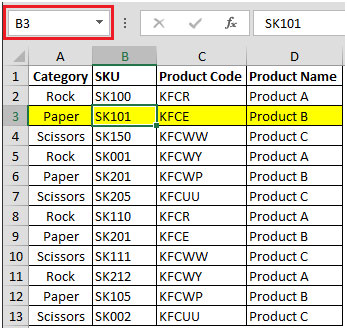Here, you can see the formula bar shows selected cell i.e. cell B3

In this way, you can highlight the rows with a single click & filter the data based on the selection of cell using VBA code.

1.•